# 2014-NIPS-Two-Stream Convolutional Networks for Action Recognition in Videos

## 3. 模型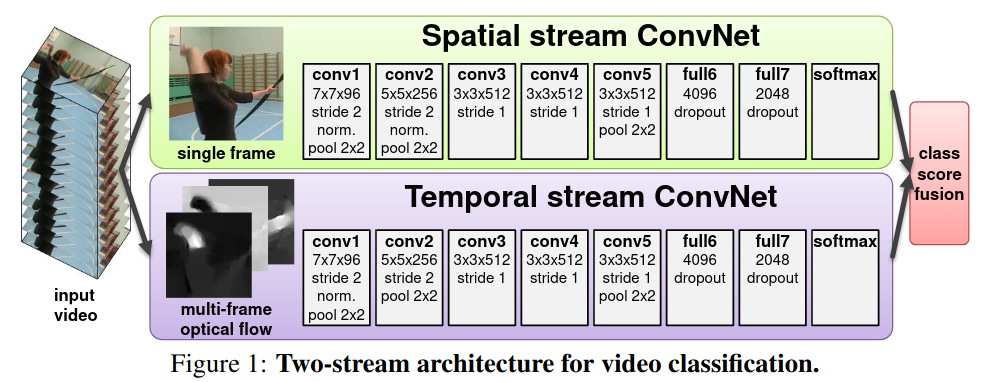• Spatial stream ConvNet（空间流网络）：类似于 AlexNet，和传统的 CNN 一样，以一系列的视频帧为输入，主要学习 apperance 信息；
• Temporal stream ConvNet（时间流网络）：以一系列视频帧的光流特征作为输入，主要学习 motion 信息；
• class score fusion：加权平均，最后再过一个 Softmax，得到最终的类别预测输出。

## 4. 方法

### 4.2 时间流网络

#### 4.2.1 光流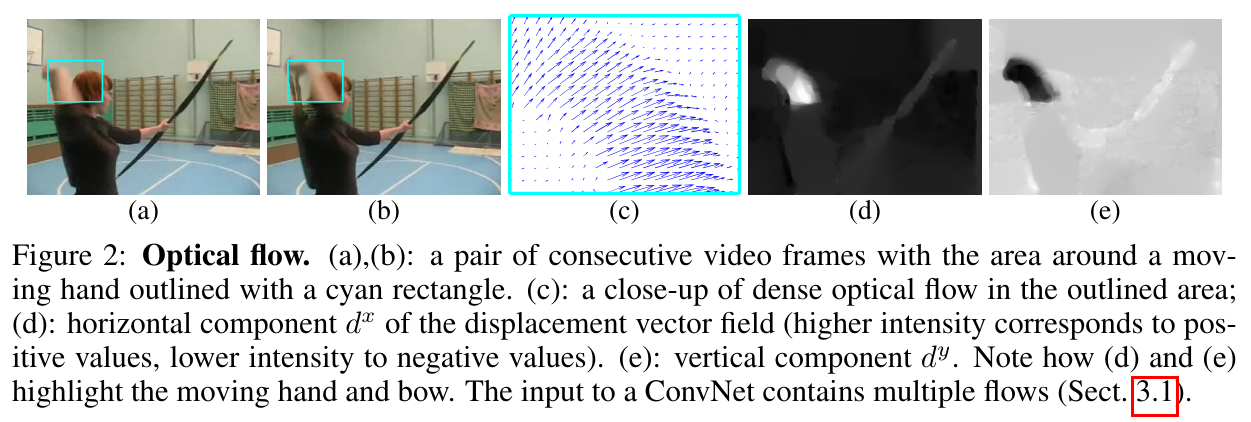#### 4.2.2 光流叠加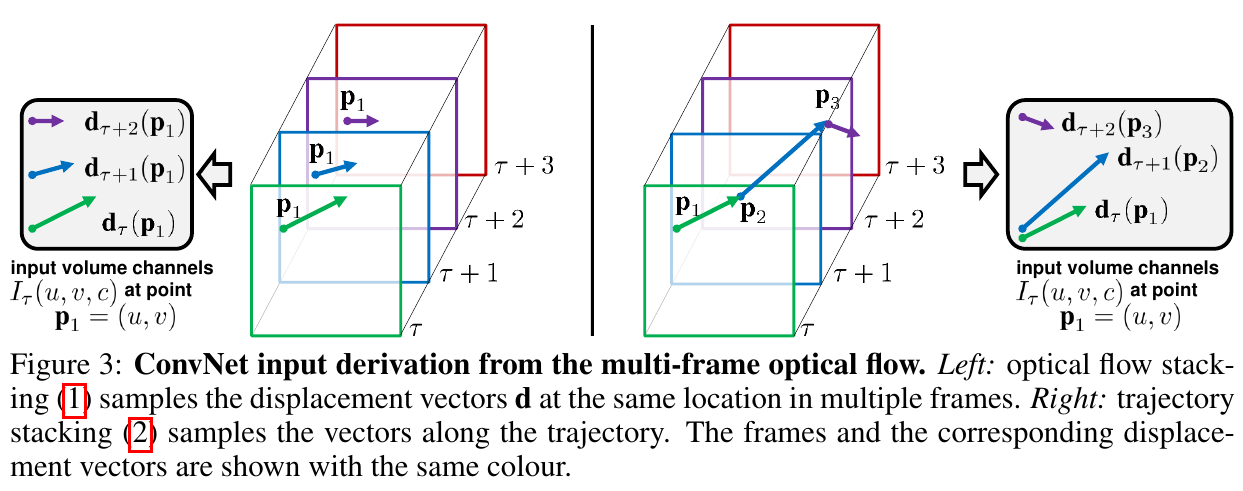• 简单的光流叠加：一种简单的拼接方式就是直接将光流图直接按照时间顺序拼接在一起，得到 $h \times w \times 2L$ 的输入张量。如 Figure 3 所示，用数学公式描述如下：

\begin{align*} &I_{\tau}(u, v, 2 k-1)=d_{\tau+k-1}^{x}(u, v) \\ &I_{\tau}(u, v, 2 k)=d_{\tau+k-1}^{y}(u, v), \quad u=[1 ; w], v=[1 ; h], k=[1 ; L] \tag{1} \end{align*}

其中，$I_\tau$ 表示拼接得到的 $h \times w \times 2L$ 的输入张量，$\boldsymbol{d}_t(u, v)$ 表示第 $t$ 帧和第 $t+1$ 帧在 $(u, v)$ 位置的光流向量，$d^x_t$$d^y_t$ 分别表示其水平和垂直方向的分量。

• 轨迹叠加：另一种方式是按照每个点光流的轨迹来叠加光流，如 Figure 3 所示，用数学公式描述如下：

\begin{align*} &I_{\tau}(u, v, 2 k-1)=d_{\tau+k-1}^{x}\left(\mathbf{p}_{k}\right) \\ &I_{\tau}(u, v, 2 k)=d_{\tau+k-1}^{y}\left(\mathbf{p}_{k}\right), \quad u=[1 ; w], v=[1 ; h], k=[1 ; L] \\ &\mathbf{p}_{1}=(u, v) ; \quad \mathbf{p}_{k}=\mathbf{p}_{k-1}+\mathbf{d}_{\tau+k-2}\left(\mathbf{p}_{k-1}\right), k>1 \tag{2} \end{align*}

## 5. 实验

### 5.1 设置

• 帧数抽取：无论视频有多才，作者都是等间距地取 $25$ 帧；
• 空间流测试输入：对于抽取的每一帧，先将其翻转增强到两帧，然后对每一帧进行 Crop（分别在四个角和中心），即得到 $10$ 帧。也就是说，原来的 $25$ 帧经过数据增强后变成 $250$ 帧作为空间流的输入，即每个视频转化为输入的张量 $250 \times h \times w \times 3$
• 时间流测试输入：对于 $25$ 帧中的每一帧，从其位置开始再在原视频中连续抽取 $11$ 帧，对其计算光流后作为时间流的输入，即每个视频转化为输入的张量为 $25 \times h \times w \times 20$
• 光流计算：作者在这篇文章中计算光流的方式是参考文献，其可以使用 GPU 加速（大概 $0.06 s$ 一张光流，但还是太慢了）。另一个问题是，存下所有光流图需要大量的存储空间，作者便将光流值 Rescale 到 $[0, 255]$ 的值，然后使用 JPEG 压缩存储光流图。

### 5.2 消融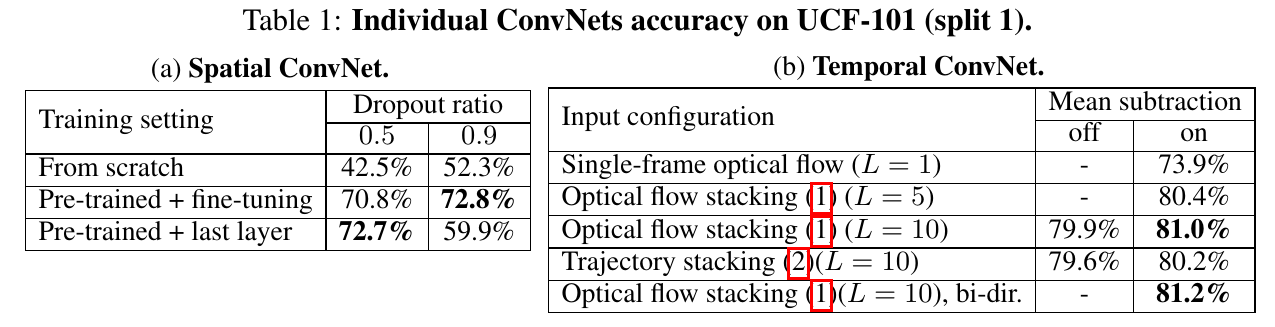### 5.3 结果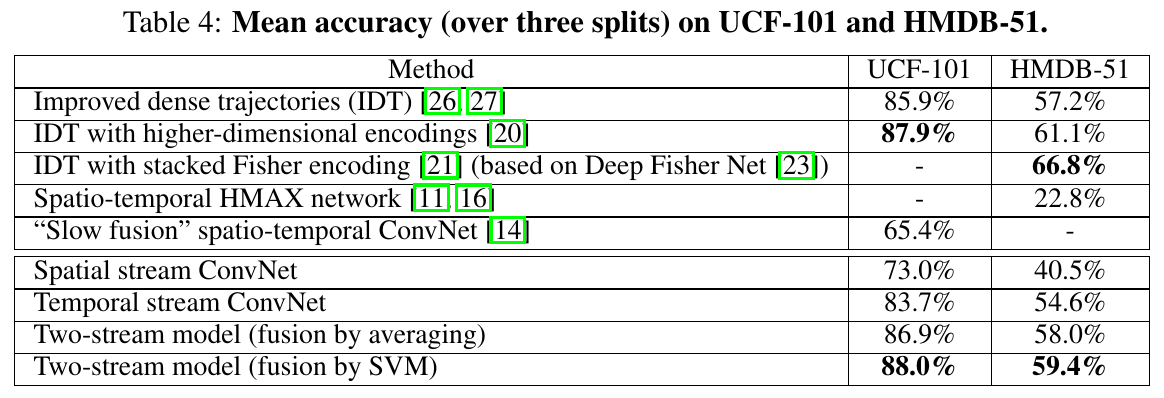## 6. 结论

• 在这篇文章中作者展出了空间流网络预训练的有效性，那对于时间流网络在大数据集上预训练会不会也要更好呢，比如 sports one million 数据集。不过光流的抽取和存储是一大挑战。
• 为什么基于轨迹的光流叠加方式不如简单的拼接，基于轨迹应该更合理才对。这在后续的工作中已经被证实，王利民老师在 CVPR2015 上就使用了基于轨迹的叠加方式，将分数刷到了 $90$ 以上。
• 视频拍摄过程中可能存在相机本身的移动，会影响光流的计算。作者提出是否可以借鉴一些传统处理相机移动的有效方法。

## 附录

1. Simonyan, K., & Zisserman, A. (2014). Two-stream convolutional networks for action recognition in videos. Advances in neural information processing systems, 27.
2. T. Brox, A. Bruhn, N. Papenberg, and J. Weickert. High accuracy optical flow estimation based on a theory for warping. In Proc. ECCV, pages 25–36, 2004.